• 1.导入numpy库 2.生成numpy数组 3.numpy的数学运算 4.numpy的N维数组表示 5.numpy的广播功能 6.numpy数组元素的访问 1.导入numpy库 import numpy as np 2.生成numpy数组 #By Bo Yang 2020.12.10 import ...
目录

1.导入numpy库
2.生成numpy数组
3.numpy的数学运算
4.numpy的N维数组表示
5.numpy的广播功能
6.numpy数组元素的访问

1.导入numpy库
import numpy as np
2.生成numpy数组
#By Bo Yang 2020.12.10
import numpy as np
x = np.array([[1,2,3],[4,5,6],[7,8,9]])

3.numpy的数学运算
#By Bo Yang 2020.12.10
import numpy as np
x = np.array([1,2,3])
y = np.array([4,5,6])
x+y #对应元素的加法
x-y #对应元素的减法
x*y #对应元素的乘法
x/y #对应元素的除法
x//y#对应元素的整除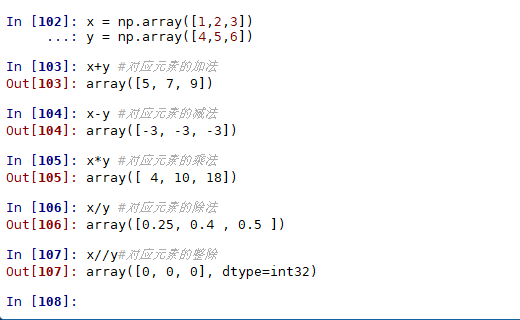4.numpy的N维数组表示
一维数组：
#By Bo Yang 2020.12.10
import numpy as np
A = np.array([1,2,3])
print(A)
A.shape #A的形状
A.dtype #矩阵A的数据类型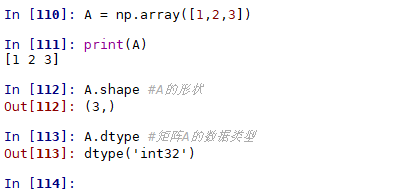二维数组：
#By Bo Yang 2020.12.10
import numpy as np
A = np.array([[1,2,3],[4,5,6]])
print(A)
A.shape #A的形状
A.dtype #矩阵A的数据类型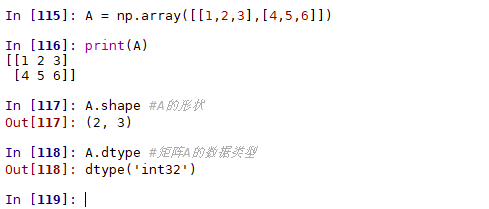#By Bo Yang 2020.12.10
import numpy as np
A = np.array([[1,2,3],[4,5,6]])
B = np.array([[1,3,5],[7,9,11]])
A + B
A - B
A * B
A / B
A //B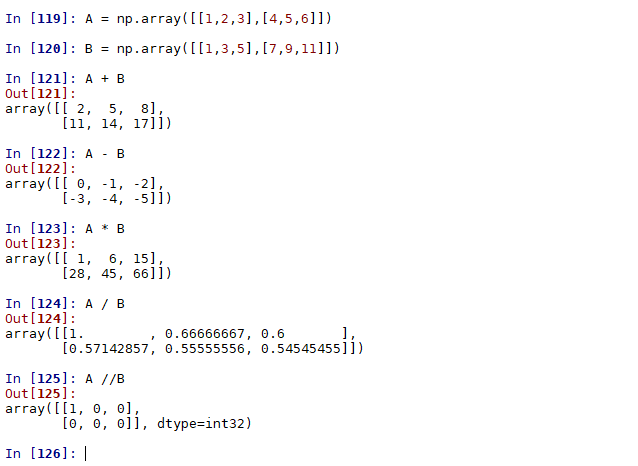5.numpy的广播功能
#By Bo Yang 2020.12.10
import numpy as np
A = np.array([1,2,3,4,5,6])
B = np.array([[1,3,5],[7,9,11]])
A*10  #numpy的广播功能
B*10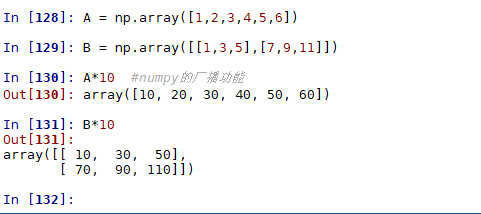6.numpy数组元素的访问
#By Bo Yang 2020.12.10
import numpy as np
A = np.array([1,2,3,4,5,6])
B = np.array([[1,3,5],[7,9,11]])
print(A)
print(B)
A
A
B #数组B的第0行
B #数组B的第1行
B
B
C = B.flatten() #将数组B转换为一维数组
C[np.array([0,2,4,5])] # 获取索引为0、2、4、5的元素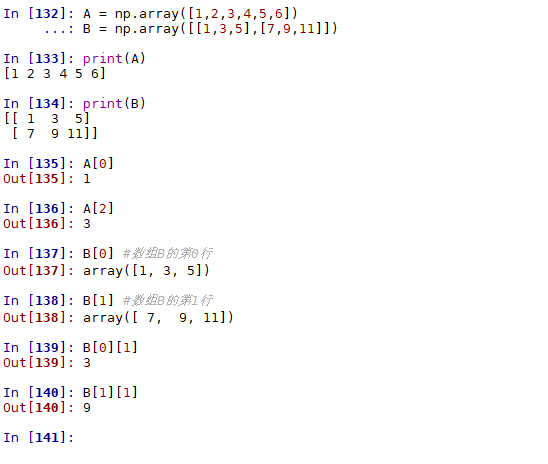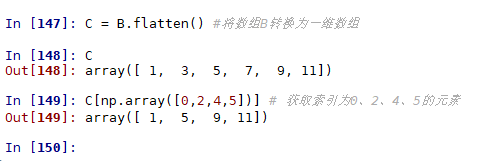展开全文• ## Python中的numpy库介绍！

万次阅读 多人点赞 2018-09-27 20:34:29
机器学习算法中大部分都是调用Numpy库来完成基础数值计算的。 安装方法： pip3 install numpy 1. ndarray数组基础 python中用列表保存一组值，可将列表当数组使用。另外，python中有array模块，但它不支持多维...
机器学习算法中大部分都是调用Numpy库来完成基础数值计算的。 安装方法：
pip3 install numpy

1. ndarray数组基础 python中用列表保存一组值，可将列表当数组使用。另外，python中有array模块，但它不支持多维数组，无论是时列表还是array模块都没有科学运算函数，不适合做矩阵等科学计算。numpy没有使用python本身的数组机制，而是提供了ndarray对象，该对象不仅能方便地存取数组，而且拥有丰富的数组计算函数。 使用前先导入Numpy模块
import numpy as np
#或
from numpy import *

1）数组的创建及使用
>>> x=np.array([[1.0,0.0,0.0],[0.,1.,2.]]) #定义了一个二维数组，大小为（2，3）
>>> x
array([[1., 0., 0.],
[0., 1., 2.]])
>>> x.ndim   #数组维度数
2
>>> x.shape    #数组的维数，返回的格式(n,m),其中n为行数，m为列数
(2, 3)
>>> x.size    #数组元素的总数
6
>>> x.dtype   #数组元素类型
dtype('float64')  #64位浮点型
>>> x.itemsize  #每个元素占有的字节大小
8
>>> x.data    #数组元素的缓冲区
<memory at 0x00000205227DAC18>

还有两种创建序列数组的函数arrange和linspace，和range函数类似，但它们都属于Numpy里面。 arange(a,b,c) 参数分别表示开始值，结束值，步长 linspace(a,b,c) 参数分别表示开始值，结束值，元素数量 还可以调用它们自身的方法reshape()指定形状
>>> arange(15).reshape(3,5)
array([[ 0,  1,  2,  3,  4],
[ 5,  6,  7,  8,  9],
[10, 11, 12, 13, 14]])
>>> arange(10,30,5)
array([10, 15, 20, 25])
>>> arange(0,2,0.3)
array([0. , 0.3, 0.6, 0.9, 1.2, 1.5, 1.8])
>>> linspace(0,2,9) # 0~2之间生成9个数字
array([0.  , 0.25, 0.5 , 0.75, 1.  , 1.25, 1.5 , 1.75, 2.  ])

还有两种创建概率分布的形式创建ndarray数组
高斯分布（正态分布）

np.random.randn(shape)：生成对应形状（shape）的高斯分布np.random.normal(loc, scale, size)：生成均值为loc，标准差为scale，形状（shape）为size的高斯分布

均匀分布

np.random.rand(shape)：生成对应形状（shape）的均匀分布np.random.uniform(low, high, size)：生成一个从[low, high)中随即采样的，样本数量为size的均匀分布

>>> a = np.random.randn(10) # 长度为10的一个一维数组
>>> a
array([ 0.12939473,  0.43128511,  1.20540157,  0.54083603,  0.80768359,
-1.24217976, -0.9713093 ,  1.43538807, -1.07227227, -1.27176462])
>>> b = np.random.normal(0, 1, (2,4)) # 均值为1，方差为0，形状为（2，4）的二维数组
>>> b
array([[ 0.4132305 , -2.06728849,  1.15189397, -1.11201615],
[ 0.39955198, -0.89664908, -0.61361683, -0.13166113]])
>>> c = np.random.rand(2,3) # 生成一个形状为（2，3）的均匀分布二维数组
>>> c
array([[0.57091351, 0.39960244, 0.77019683],
[0.11316102, 0.59354993, 0.37849038]])
>>> d = np.random.uniform(-1,1,10)
>>> d
array([-0.34374858, -0.27026865,  0.27073922, -0.42654097, -0.38736897,
0.16293278, -0.79578655, -0.04825995,  0.28444576,  0.99118406])

2）特殊数组
zeros数组：全零数组，元素全为零。ones数组：全1数组，元素全为1。empty数组：空数组，元素全近似为0。
>>> zeros((3,4))
array([[0., 0., 0., 0.],
[0., 0., 0., 0.],
[0., 0., 0., 0.]])
>>> ones((2,3,4),dtype=int16)
array([[[1, 1, 1, 1],
[1, 1, 1, 1],
[1, 1, 1, 1]],

[[1, 1, 1, 1],
[1, 1, 1, 1],
[1, 1, 1, 1]]], dtype=int16)
>>> empty((5,3))
array([[6.23042070e-307, 1.42417221e-306, 1.37961641e-306],
[1.11261027e-306, 1.11261502e-306, 1.42410839e-306],
[7.56597770e-307, 6.23059726e-307, 1.42419530e-306],
[7.56599128e-307, 1.11260144e-306, 6.89812281e-307],
[2.22522596e-306, 2.22522596e-306, 2.56761491e-312]])

3）数组索引 Numpy数组每个元素，每行元素，每列元素都可以用索引访问。
>>> c=arange(24).reshape(2,3,4) # reshape()改变数组形状
>>> print(c)
[[[ 0  1  2  3]
[ 4  5  6  7]
[ 8  9 10 11]]

[[12 13 14 15]
[16 17 18 19]
[20 21 22 23]]]
>>> print(c[1,2,:])
[20 21 22 23]
>>> print(c[0,1,2])
6

4）数组运算
算术运算：数组的加减乘除以及乘方运算方式为，相应位置的元素分别进行运算。
>>> a=array([20,30,40,50])
>>> aa=arange(1,5)
>>> a/aa
array([20.        , 15.        , 13.33333333, 12.5       ])
>>> b=arange(4)
>>> b
array([0, 1, 2, 3])
>>> c=a-b
>>> c
array([20, 29, 38, 47])
>>> b**2
array([0, 1, 4, 9], dtype=int32)
>>> A=array([[1,1],[0,1]])
>>> b=array([[2,0],[3,4]])
>>> A*b
array([[2, 0],
[0, 4]])
>>> A.sum()
3
>>> A.min()
0
>>> A.max()
1

逻辑运算
arr > a : 返回arr中大于a的一个布尔值数组
arr[arr>a] : 返回arr中大于a的数据构成的一维数组
np.all(): 括号内全为真则返回真，有一个为假则返回false
np.any() : 括号内全为假则返回假，有一个为真则返回真
np.where(): 三元预算符, 判断同时赋值
如：np.where(arr>0, 1, 0)
复合逻辑运算：
与：np.logical_and(): 括号为一系列表达式
或：np.logical_or()

统计运算
统计指标函数：min, max, mean, median, var, std
np.函数名
ndarray.方法名
axis参数：axis=0代表列，axis=1代表行
最大值最小值的索引函数：
np.argmax(arr, axis=)
np.argmin(arr, axis=)

5）数组的拷贝 数组的拷贝分浅拷贝和深拷贝两种，浅拷贝通过数组变量的赋值完成，深拷贝使用数组对象的copy方法。 浅拷贝只拷贝数组的引用，如果对拷贝进行修改，源数组也将修改。如下：
>>> a=ones((2,3))
>>> a
array([[1., 1., 1.],
[1., 1., 1.]])
>>> b=a
>>> b[1,2]=2
>>> a
array([[1., 1., 1.],
[1., 1., 2.]])
>>> b
array([[1., 1., 1.],
[1., 1., 2.]])

深拷贝会复制一份和源数组一样的数组，新数组与源数组会存放在不同内存位置，因此对新数组的修改不会影响源数组。如下：
>>> a=ones((2,3))
>>> b=a.copy()
>>> b[1,2]=2
>>> a
array([[1., 1., 1.],
[1., 1., 1.]])
>>> b
array([[1., 1., 1.],
[1., 1., 2.]])

6）广播机制 numpy中不同维度的数组是可以进行算数运算的，只要满足广播机制即可。
广播机制: 1.数组拥有相同形状-->（3，4）+（3，4）
2.当前维度相等--->（3，4）+（4，）
3.当前维度有一个是1--->(3,1,5)+(1,3,5)

7）合并与分割 np.hstack((a,b))：按行合并，要求a和b的

行

数

相

同

\color{red}{行数相同}

np.vstack((a,b))：按列合并，要求a和b的

列

数

相

同

\color{red}{列数相同}

np.c_[a,b]：用法如同np.hstack((a,b)) np.r_[a,b]：用法如同np.vstack((a,b))
>>> a = np.array([1,2,3])
>>> b = np.array([4,5,6])
>>> a,b
(array([1, 2, 3]), array([4, 5, 6]))
>>> np.hstack((a,b))
array([1, 2, 3, 4, 5, 6])
>>> np.vstack((a,b))
array([[1, 2, 3],
[4, 5, 6]])

np.concatenate((a,b), axis = 1)：按行合并，要求a和b的行数相同 np.concatenate((a,b), axis = 0)：按列合并，要求a和b的列数相同 注意：

如

果

不

指

定

a

x

i

s

，

则

默

认

a

x

i

s

=

0

，

即

按

列

合

并

。

并

且

一

维

数

组

只

能

按

行

合

并

\color{red}{如果不指定axis，则默认axis=0，即按列合并。并且一维数组只能按行合并}

>>> a = np.array([1,2,3]
>>> b = np.array([4,5,6])
>>> np.concatenate((a,b),axis=0)
array([1, 2, 3, 4, 5, 6])
>>> np.concatenate((a,b),axis=1) # a，b都是一维数组，只能按axis=0合并
Traceback (most recent call last):
File "<stdin>", line 1, in <module>
numpy.AxisError: axis 1 is out of bounds for array of dimension 1
>>> x = np.array([[1,2],[3,4]])
>>> y = np.array([[5,6]])
>>> np.concatenate((x,y),axis=0)
array([[1, 2],
[3, 4],
[5, 6]])
>>> np.concatenate((x,y.T),axis=1) # y.T表示将y数组转置
array([[1, 2, 5],
[3, 4, 6]])

np.split(arr, n)：n要么是整数，要么是列表，用来进行划分，n为整数时必须是能均匀划分 np.array_split(arr, n)：类似上面的用法，但是可以不均等划分
>>> x = np.arange(9.0)
>>> np.split(x, 3)
[array([ 0.,  1.,  2.]), array([ 3.,  4.,  5.]), array([ 6.,  7.,  8.])]
>>> x = np.arange(8.0)
>>> np.split(x, [3, 5, 6, 10])
[array([ 0.,  1.,  2.]),
array([ 3.,  4.]),
array([ 5.]),
array([ 6.,  7.]),
array([], dtype=float64)]

>>> x = np.arange(8.0)
>>> np.array_split(x, 3)
[array([ 0.,  1.,  2.]), array([ 3.,  4.,  5.]), array([ 6.,  7.])]

>>> x = np.arange(7.0)
>>> np.array_split(x, 3)
[array([ 0.,  1.,  2.]), array([ 3.,  4.]), array([ 5.,  6.])]

8）numpy降维 ravel()：返回一维数组，但是改变返回的一维数组内容后，原数组的值也会相应改变 flatten()：返回一维数组，改变返回的数组不影响原数组
>>> a
array([[1, 2, 3],
[7, 8, 9]])
>>> b
array([[4, 5, 6],
[1, 2, 3]])
>>> c = a.ravel()
>>> c
array([1, 2, 3, 7, 8, 9])
>>> d = b.flatten()
>>> d
array([4, 5, 6, 1, 2, 3])
>>> c=100
>>> c
array([100,   2,   3,   7,   8,   9])
>>> a
array([[100,   2,   3],
[  7,   8,   9]])
>>> d=100
>>> d
array([[100, 100],
[  6,   1],
[  2,   3]])
>>> b
array([[4, 5, 6],
[1, 2, 3]])


2. 矩阵 1）创建矩阵 Numpy的矩阵对象与数组对象相似，主要不同之处在于，矩阵对象的计算遵循矩阵数学运算规律。矩阵使用matrix函数创建。
>>> A=matrix('1.0 2.0;3.0 4.0')
>>> A
matrix([[1., 2.],
[3., 4.]])
>>> b=matrix([[1.0,2.0],[3.0,4.0]])
>>> b
matrix([[1., 2.],
[3., 4.]])
>>> type(A)
<class 'numpy.matrixlib.defmatrix.matrix'>

2）矩阵运算 矩阵的常用数学运算有转置，乘法，求逆等。
>>> A.T      #转置
matrix([[1., 3.],
[2., 4.]])
>>> x=matrix('5.0 7.0')
>>> y=x.T
>>> y
matrix([[5.],
[7.]])
>>> print(A*y)   #矩阵乘法
[[19.]
[43.]]
>>> print(A.I)   #逆矩阵
[[-2.   1. ]
[ 1.5 -0.5]]


3.Numpy线性代数相关函数
numpy.dot() 此函数返回两个数组的点积。 对于二维向量，其等效于矩阵乘法。 对于一维数组，它是向量的内积。 对于 N 维数组，它是a的最后一个轴上的和与b的倒数第二个轴的乘积。
>>> a=np.array([[1,2],[3,4]])
>>> b=np.array([[11,12],[13,14]])
>>> np.dot(a,b)
array([[37, 40],     #[[1*11+2*13, 1*12+2*14],[3*11+4*13, 3*12+4*14]]
[85, 92]])

numpy.vdot() 此函数返回两个向量的点积。 如果第一个参数是复数，那么它的共轭复数会用于计算。 如果参数id是多维数组，它会被展开。
>>> np.vdot(a,b)
130    #1*11+2*12+3*13+4*14=130

numpy.inner() 此函数返回一维数组的向量内积。 对于更高的维度，它返回最后一个轴上的和的乘积。
>>> x=np.array([1,2,3])
>>> y=np.array([0,1,0])
>>> print(np.inner(x,y))
2      # 等价于 1*0+2*1+3*0

numpy.matmul() 函数返回两个数组的矩阵乘积。 虽然它返回二维数组的正常乘积，但如果任一参数的维数大于2，则将其视为存在于最后两个索引的矩阵的栈，并进行相应广播。 另一方面，如果任一参数是一维数组，则通过在其维度上附加 1 来将其提升为矩阵，并在乘法之后被去除。
#对二维数组（列表），就相当于矩阵乘法
>>> a=[[1,0],[0,1]]
>>> b=[[4,1],[2,2]]
>>> print(np.matmul(a,b))
[[4 1]
[2 2]]
#二维和一维运算
>>> a=[[1,0],[0,1]]
>>> b=[1,2]
>>> print(np.matmul(a,b))
[1 2]
>>> print(np.matmul(b,a))
[1 2]
#维度大于2的
>>> a=np.arange(8).reshape(2,2,2)
>>> b=np.arange(4).reshape(2,2)
>>> print(np.matmul(a,b))
[[[ 2  3]
[ 6 11]]

[[10 19]
[14 27]]]

numpy.linalg.det() 行列式在线性代数中是非常有用的值。 它从方阵的对角元素计算。 对于 2×2 矩阵，它是左上和右下元素的乘积与其他两个的乘积的差。 换句话说，对于矩阵[[a，b]，[c，d]]，行列式计算为ad-bc。 较大的方阵被认为是 2×2 矩阵的组合。 numpy.linalg.det()函数计算输入矩阵的行列式。
>>> a=np.array([[1,2],[3,4]])
>>> print(np.linalg.det(a))
-2.0000000000000004
>>> b=np.array([[6,1,1],[4,-2,5],[2,8,7]])
>>> print(b)
[[ 6  1  1]
[ 4 -2  5]
[ 2  8  7]]
>>> print(np.linalg.det(b))
-306.0
>>> print(6*(-2*7-5*8)-1*(4*7-5*2)+(4*8- -2*2))
-306

numpy.linalg.solve() 该函数给出了矩阵形式的线性方程的解。 例： x + y + z = 6 2y + 5z = -4 2x + 5y - z = 27 写成矩阵形势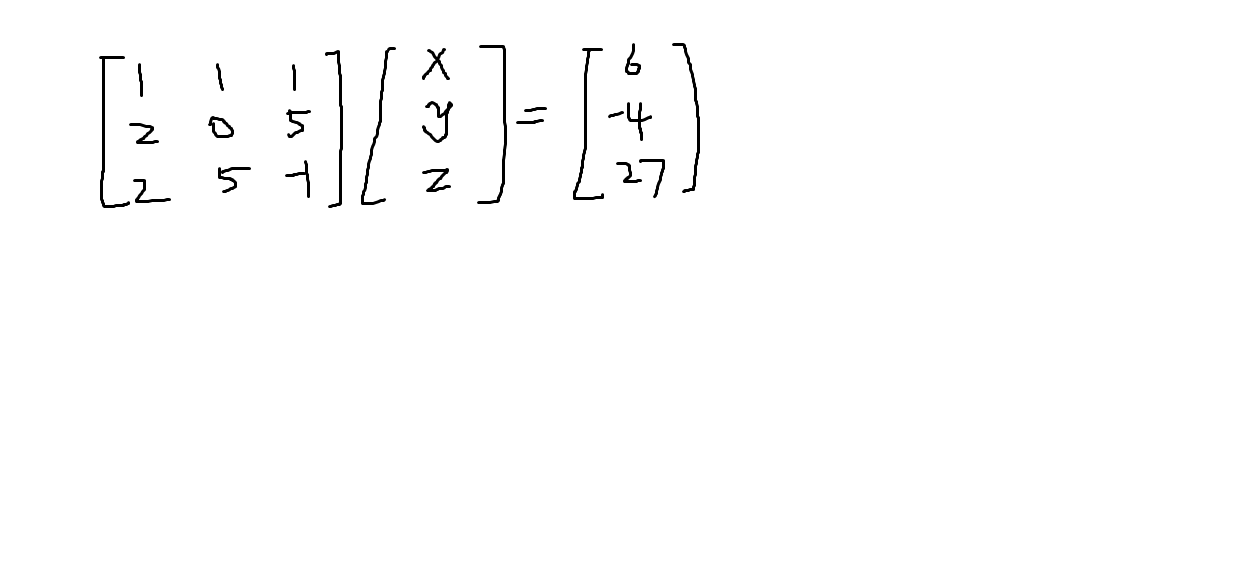可表示为AX=B 即求X=A^(-1)B 逆矩阵可以用numpy.linalg.inv()函数来求
>>> x=np.array([[1,2],[3,4]])
>>> y=np.linalg.inv(x)
>>> x
array([[1, 2],
[3, 4]])
>>> y
array([[-2. ,  1. ],
[ 1.5, -0.5]])
>>> np.dot(x,y)
array([[1.0000000e+00, 0.0000000e+00],
[8.8817842e-16, 1.0000000e+00]])

计算线性方程的解
a=np.array([[1,1,1],[0,2,5],[2,5,-1]])
print('数组a:')
print(a)
ainv=np.linalg.inv(a)
print('a的逆矩阵')
print(ainv)
print('矩阵b:')
b=np.array([,[-4],])
print(b)
print('计算：A^(-1)B:')
x=np.linalg.solve(a,b)
print(x)

输出如下：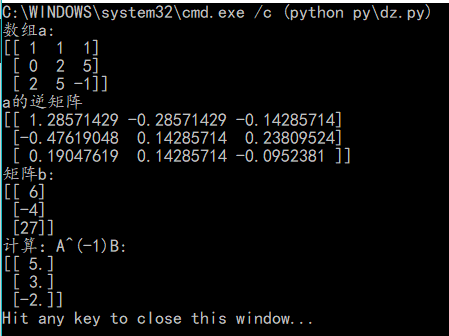完毕！
展开全文• NumPy是一个开源的Python科学计算基础，包含： • 一个强大的N维数组对象 ndarray • 广播功能函数 • 整合C/C++/Fortran代码的工具 • 线性代数、傅里叶变换、随机数生成等功能 NumPy是SciPy、Pandas等数据处理或...
NumPy
NumPy是一个开源的Python科学计算基础库，包含： • 一个强大的N维数组对象 ndarray • 广播功能函数 • 整合C/C++/Fortran代码的工具 • 线性代数、傅里叶变换、随机数生成等功能 NumPy是SciPy、Pandas等数据处理或科学计算库的基础
NumPy的引用
import numpy as np
N维数组对象：ndarray
ndarray是一个多维数组对象，由两部分构成： • 实际的数据 • 描述这些数据的元数据（数据维度、数据类型等） ndarray数组一般要求所有元素类型相同（同质），数组下标从0开始
ndarray对象的属性 .ndim 秩，即轴的数量或维度的数量 .shape ndarray对象的尺度，对于矩阵，n行m列 .size ndarray对象元素的个数，相当于.shape中n*m的值 .dtype ndarray对象的元素类型 .itemsize ndarray对象中每个元素的大小，以字节为单位
ndarray数组的创建方法
•从Python中的列表、元组等类型创建ndarray数组 • 使用NumPy中函数创建ndarray数组，如：arange, ones, zeros等 • 从字节流（raw bytes）中创建ndarray数组 • 从文件中读取特定格式，创建ndarray数组
1）从Python中的列表、元组等类型创建ndarray数组
x= np.array(list/tuple)
x= np.array(list/tuple, dtype=np.float32)
当np.array()不指定dtype时，NumPy将根据数据情况关联一个dtype类型
2）使用NumPy中函数创建ndarray数组，如：arange, ones, zeros等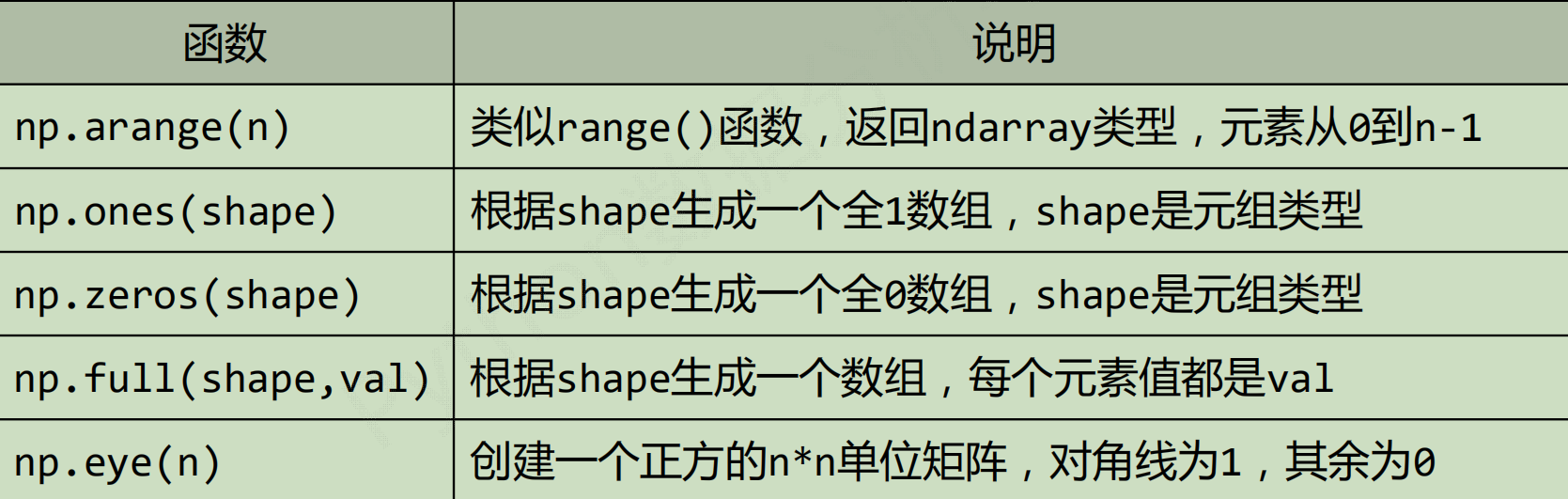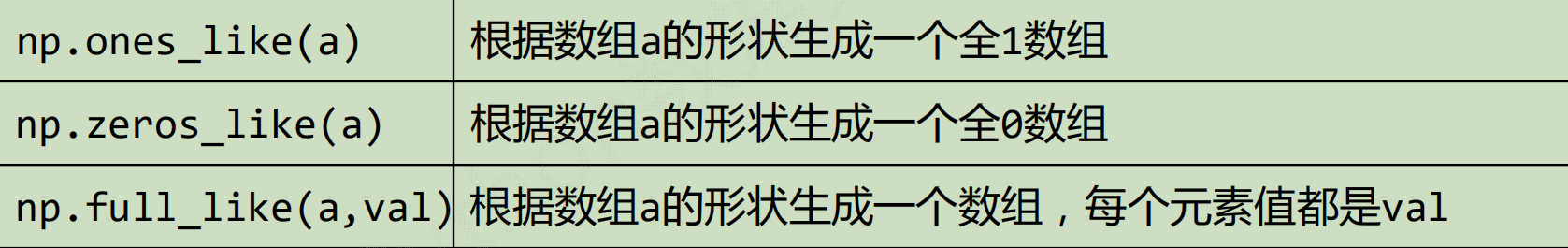3）使用NumPy中其他函数创建ndarray数组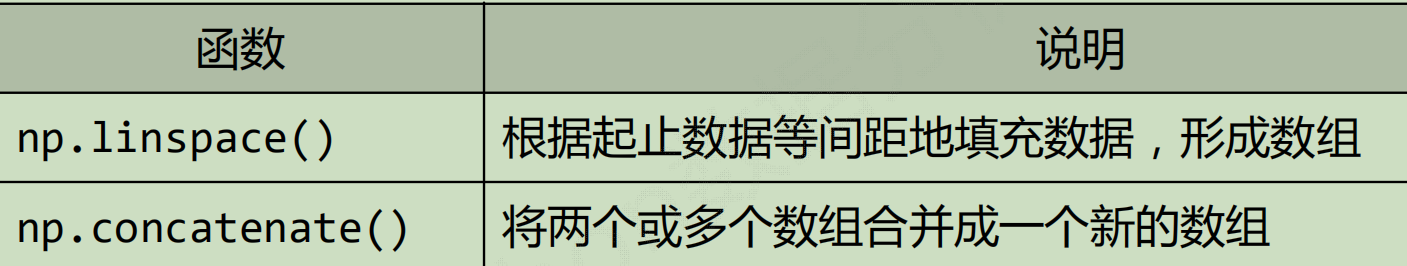ndarray数组的变换
对于创建后的ndarray数组，可以对其进行维度变换和元素类型变换
a= np.ones((2,3,4), dtype=np.int32
维度变换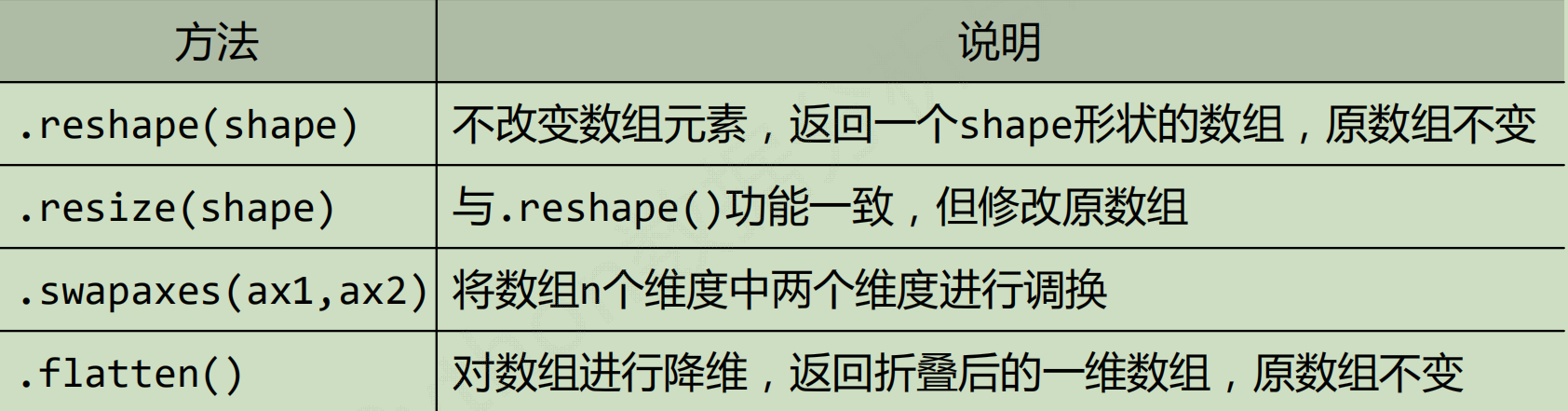类型变换
new_a = a.astype(new_type)

astype()方法一定会创建新的数组（原始数据的一个拷贝），即使两个类型
ndarray数组向列表的转换
ls= a.tolist

ndarray数组的操作
数组的索引和切片 索引：获取数组中特定位置元素的过程 切片：获取数组元素子集的过程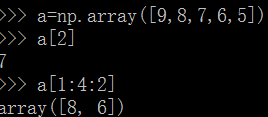起始编号: 终止编号(不含): 步长，3元素冒号分割，编号0开始从左递增，或‐1开始从右递减
ndarray数组的运算
数组与标量之间的运算作用于数组的每一个元素
NumPy一元函数
对ndarray中的数据执行元素级运算的函数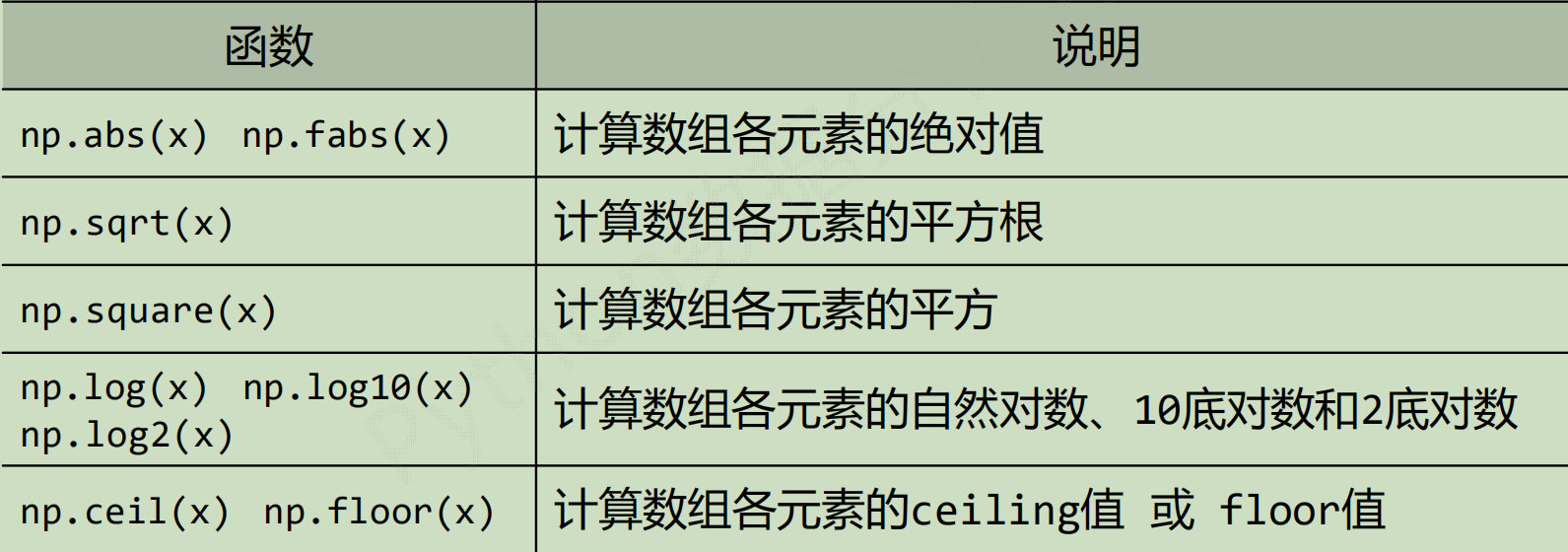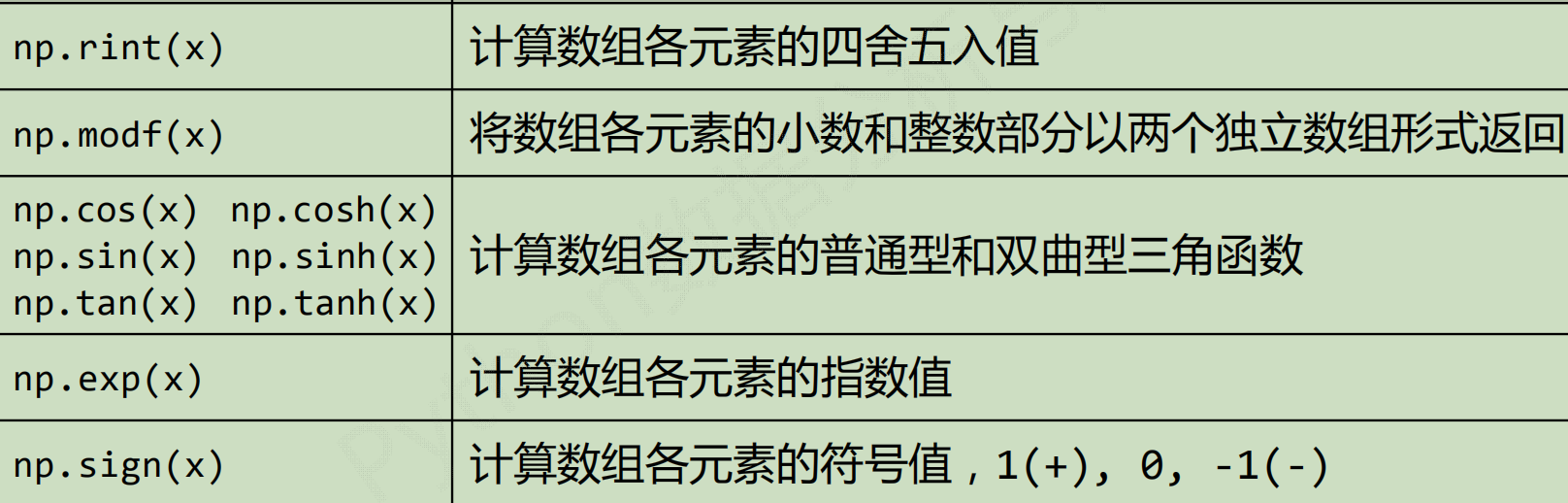floor(x)返回的是小于或等于x的最大整数
ceil(x)返回的是大于x的最小整数。
NumPy二元函数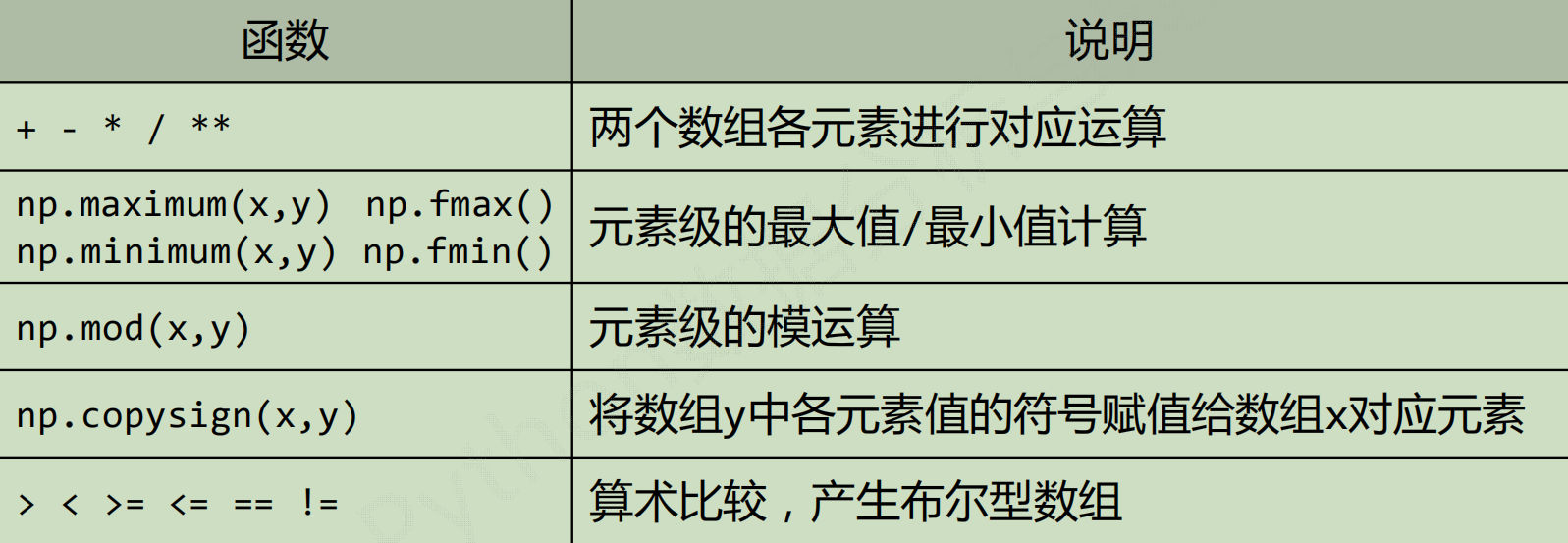展开全文• numpy库简介 numpy是一个开源的python科学计算基数库，包含： 一个强大的N为数组对象ndarray 广播功能函数 整合C/C++/Fortran代码工具 线性代数，傅里叶变换，随机数生成等功能 numpy是scipy、pandas等数据...
numpy库简介
numpy是一个开源的python科学计算基数库，包含：
一个强大的N为数组对象ndarray广播功能函数 整合C/C++/Fortran代码工具线性代数，傅里叶变换，随机数生成等功能
numpy是scipy、pandas等数据处理或科学计算库的基础。
Numpy库的引用
    inport numpy as np

N维数组对象 ndarray
Ndarray是一个多维数组对象，由两部分构成：
实际数据描述这些数据的元数据（数据维度，数据类型，等）Ndarray数组一般要求所有元素类型相同（同质），数组下标从0开始
Np.array()输出成[ ]元素由空格分割。 轴（axis）：保存数据的维度， 秩（rank）：轴的数量
Ndarray实例
import numpy as np
pa = [0, 1, 2, 3, 6]
pb = [4, 2, 5, 6, 8]
def psm():
pc = []
for i in range(len(pa)):
pc.append(pa[i]**2+pb[i]**3)
return pc
def nsm():
na = np.array(pa)
nb = np.array(pb)
nc = na**2+nb**3
return nc
print(psm())
print(nsm())
[64, 9, 129, 225, 548]
[ 64   9 129 225 548]

Ndarray对象的属性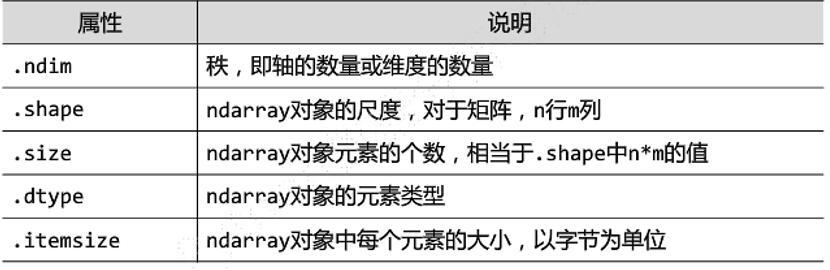import numpy as np
pa = [0, 1, 2, 3, 6]
pb = [4, 2, 5, 6, 8]
a=np.array([pa,pb])
print(a)
[[0 1 2 3 6]
[4 2 5 6 8]]
print(a.ndim)   #输出轴的数量或维度的数量
2
print(a.shape)  #对象的尺度，对于矩阵，n行m列
(2, 5)
print(a.size)   #对象元素的个数，相当于对象中m*n的值
10
print(a.dtype)  #对象的元素类型
int32
print(a.itemsize) #对象中每个元素的大小，一字节为单位
4

Ndarray的元素类型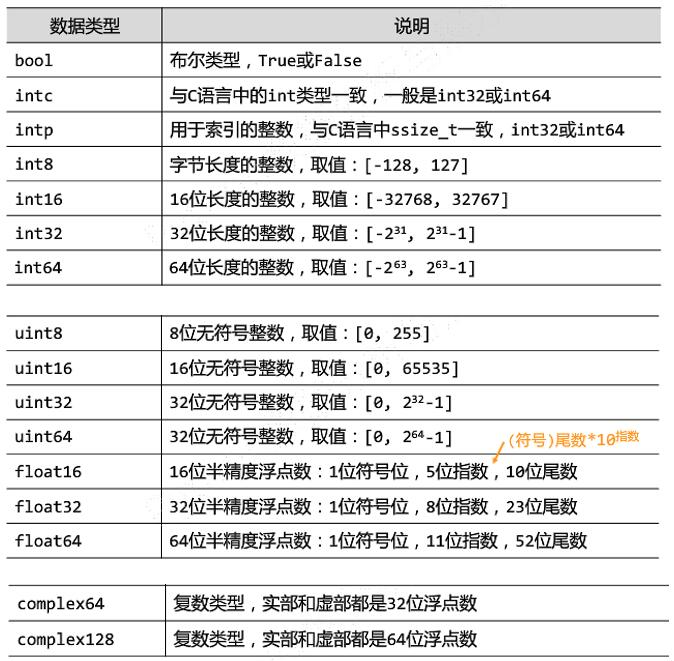非同质的ndarray对象
Ndarray数组可以有非同质对象构成 非同质ndarray元素为对象类型， 非同质ndarray对象无法有效发挥numpy优势，尽量避免使用。
import numpy as np
pa = [0, 1, 2, 3, 6]
pb = [4, 2, 5, 6]
a=np.array([pa,pb])
print(a)
[list([0, 1, 2, 3, 6]) list([4, 2, 5, 6])]
print(a.ndim)   #输出轴的数量或维度的数量
1
print(a.shape)  #对象的尺度，对于矩阵，n行m列
(2,)
print(a.size)   #对象元素的个数，相当于对象中m*n的值
2
print(a.dtype)  #对象的元素类型
object
print(a.itemsize) #对象中每个元素的大小，一字节为单位
8

展开全文python
• 主要介绍了Python Numpy库安装与基本操作,简单介绍了Numpy库的基本功能、并结合实例形式分析了基于Numpy库的数组与矩阵相关操作技巧,需要的朋友可以参考下
• 主要介绍了Python Numpy库常见用法,结合实例形式详细Fenix了Python numpy库基本功能、原理以及数组常见操作技巧,需要的朋友可以参考下
• 介绍numpy库，数组的基本属性、索引切片、运算、重塑等内容np
• ## python 如何安装numpy库？

万次阅读 多人点赞 2019-01-10 21:59:06
） 首先我们要找到python安装的位置 win+R打开 进入以后输入： where python 找到安装目录后，找到Scripts文件夹所在位置：如Programs\Python\Python36\Scripts 去https://pypi.org/project/numpy/#files下载对应...
• 主要介绍了Python NumPy库安装使用笔记,本文讲解了NumPy的安装和基础使用,并对每一句代码都做了详细解释,需要的朋友可以参考下
• 主要介绍了numpy库reshape用法详解，文中通过示例代码介绍的非常详细，对大家的学习或者工作具有一定的参考学习价值，需要的朋友们下面随着小编来一起学习学习吧
• 主要介绍了Python Numpy库datetime类型的处理详解，Python中自带的处理时间的模块就有time 、datetime、calendar，另外还有扩展的第三方库，如dateutil等等。。当我们用NumPy库做数据分析时，如何转换时间呢？需要的...
• ## Python Numpy库教程

万次阅读 多人点赞 2018-06-02 15:10:47
本章节主要介绍的是python的一个常用库numpy，通过本章教程希望能够掌握numpy库一些常用的方法。 简介 NumPy 是一个 Python 的第三方库，代表 “Numeric Python”，主要用于数学/科学计算。 它是一个由多维数组...python 机器学习 人工智能
• Python 中的numpy 　ipynb 格式
• 导入Numpy库，并命名为np：2.查看版本3.通过列表创建一维数组：4.通过列表创建二维数组：5.创建全为0的二维数组6.创建全为1的三维数组7.创建一维等差数组8. 创建二维等差数组：9. 创建单位矩阵（二维数组）：10. ...python
• 安装numpy库的方法 win+R，输入cmd，打开cmd 在cmd中输入pip install numpy，回车 输入pip list，查看numpy是否安装成功 4.在cmd中输入python，回车，在输入import numpy， 验证numpy库是否可以被调用，没有...
• 安装numpy库： pip install -i https://pypi.doubanio.com/simple/ --trusted-host pypi.doubanio.com pillow （以下是废话，qaq.....，安装不成功再往下看） 昨天为了实现对一张照片灰度化处理然后成为素描化的...
•python
• Numpy库基本使用Numpy库基本使用Numpy库基本使用 1 为什么要用Numpy 1.1  低效的Python for循环 【例】 求100万个数的倒数 def compute_reciprocals(values): res = [] for value in values: # 每遍历到一个元素...
• python的Numpy库基础一.X = np.array(z)二.索引切片X[x1:x2,y1:y2,:,……]三. Y = X[x1:x2,y1:y2,:,……] 条件表达式的使用 Numpy库 NumPy(Numerical Python) 是 Python 语言的一个扩展程序库，python本事就携带了......